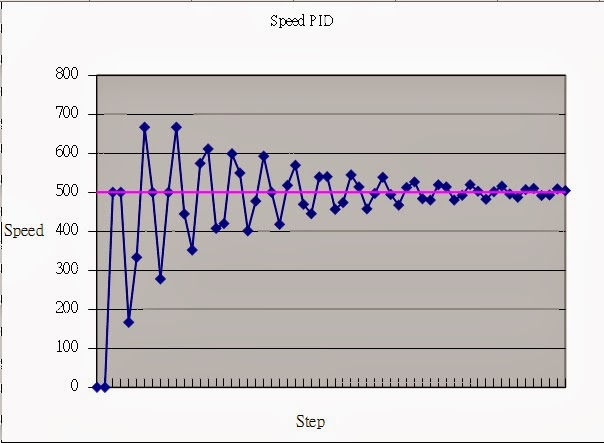## 2013年11月30日 星期六

### PID Control 精彩討論 (2) ─ 微分項與負載Kp = 1, Ki = 1, Kd = 1, T = 3

Q1：

A1：
P 控制或 PD 控制因為缺積分項有偏差問題，速度控制確定是不適用，但可用於位置控制。PID 一般是用來解決負載變動問題，

Q2：

A2：

1. T=1, Kp=1, Ki=1, Kd=1
2. T=10, Kp=1, Ki=1, Kd=1
3. T=10, Kp=1, Ki=1, Kd=2
4. T=10, Kp=1, Ki=1, Kd=0
5. T=1, Kp=0.4, Ki=0.4, Kd=0
6. T=10, Kp=0.4, Ki=0.4, Kd=0
7. T=10, Kp=1, Ki=1, Kd= -1

Q3：

T=10, Kp=1, Ki=1, Kd=1

T=1,Kp=0.1, Ki=0.1, Kd=0.1

1. T=1, Kp=1, Ki=1, Kd=1 : 因PID參數值過大造成系統補償過頭(無法補償)而發散。
2. T=10, Kp=1, Ki=1, Kd=1 : 負載進入系統後可抑制(輸出響應變慢)PID參數，使系統慢慢達到設定目標。
3. T=10, Kp=1, Ki=1, Kd=2 : 增加D(t)的補償量，但會出現第一個震盪點(Step 4)。要怎樣消除此現象?(週期出現，但後續因I(t)變大而使D(t)影響變小)
4. T=10, Kp=1, Ki=1, Kd=0 : 靠I(t)將系統穩態誤差縮小(達到目標)。
5. T=1, Kp=0.4, Ki=0.4, Kd=0 : 負載移除並縮小PI參數，避免補償過大造成系統發散。
6. T=10, Kp=0.4, Ki=0.4, Kd=0 : 增加負載時系統更緩慢(因PI參數值小)到達目標值。
7. T=10, Kp=1, Ki=1, Kd= -1 : Kd為負值時可抑制Kp與Ki得效果，但Kd最小不可為負的Kp+Ki(例:Kp=1,Ki=1,Kd=-2)，否則系統一開始會有U(t)為負數值得風險。

當系統負載進入系統後會使響應變慢(出力變小，緩慢到達目標值)。增加Kp值可使得U(t)的初始(前幾個Step)補償值增加，後續因誤差值E(t)變小使P(t)對於U(t)貢獻度變小。增加Ki可以系統快速累積I(t)而達到R(t)，但Ki如果過大時會造成系統Overshoot。增加Kd可使得U(t)的初始(前幾個Step)補償值減少(抑制正速度變化)，誤差變化率(加速度)越小則代表越接近R(t)，D(t)對U(t)的影響也就越小。

Kp與Kd都是屬於暫態響應的變數，只在系統一開始(前幾個Step)會有影響，後續則因為I(t)的累加使數值變大而由Ki的參數去主導U(t)的趨勢，所以Ki屬於穩態變數。一般的設計用PI設計就夠了。如有考慮速度變化時才需使用Kd。當負載進入時是可以使原本會發散的系統變成穩定。

A3：

T=10, Kp=1, Ki=1, Kd=1，可以直接從 T 係數看到負載加重，而
T=1,Kp=0.1, Ki=0.1, Kd=0.1，比較難看出，

Power = U / 8 / T [1 + (T-1)/15]

T=10, Kp=1, Ki=1, Kd=2，震盪點是消不掉的，Kd 是用來抑制控制初期的變動，

Q4：

A4：

T=1, Kp=Ki=0.4, Kd=0, 穩定時 U 大約為 500,
power = U / 8 / T * [1 + (T-1)/15] = U / 8 / 1 * 1 = U / 8 與原公式相同
T=10, Kp=Ki=Kd=1, 穩定時 U 大約為 5000 ~= 500 * T,
power = U / 8 / T * [1 + (T-1)/15] = U / 8 / 10 * 1.6 = 5000 / 80 * 1.6 = 100

Q5：

Power = 100 = 6000 / 80 * [1 + (10-1)/X]

X~=27。

A5：

Q6：

A6：

Q7：

A7：

Q8：

A8：